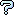All about flooble | fun stuff | Get a free chatterbox | Free JavaScript | Avatarsperplexus dot infoThree Periodical Questions (Posted on 2006-10-16)Assume X is a positive integer. If you divide 1/X, you will get a number that eventually becomes periodic: 1/9= 0.111..., 1/4= 0.25000..., and so on. Let's call numbers like 1/9 "pure" periodic, since the fractional part is formed just by the periodic part.

Prove that:

1. For all X, you will get a periodic part, and its length will be less than X.

2. If X is even, 1/X cannot be "pure". What happens if X is odd?

3. For some X, 1/X is "pure", the period length is even, and you can split the period in two halves that sum up to all nines. For example, 1/7=0.142857 142857... and 142+857=999. Which are these X values?

 See The Solution Submitted by Federico Kereki Rating: 3.0000 (1 votes)Comments: ( Back to comment list | You must be logged in to post comments.)What is 'pure'? (and part 1 & 2))| Comment 1 of 4

Am is understanding the 'pure' concept?
1/6 is not pure because the 1 before the repeating 6's?  .1666...
1/33 is pure because the are no digits before the repeating 03?  .030303...

Part 1 is simple enough.  If you divide 1 by X you get a remainder after figuring each digit.  Eventually you have to repeat one.  There are only X-1 digits that have not been used already (1 is used at the beinning) so once you get one of these numbers you will repeat.

Part 2 I can see but not prove yet:
The number will be pure if it does not have 2 or 5 as a factor.
It also appears that whichever number of 2's or 5's appears most in the factorization of X is the number of digits before the repeated part begins.

For example 120= 2^3*3*5 which has three 2's but only one 5.
1/120 leads with 3 unrepeated digits: .008333...

 Posted by Jer on 2006-10-16 11:09:06Please log in:

 Search: Search body:
Forums (0)